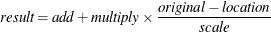# The STDIZE Procedure

## Overview: STDIZE Procedure

The STDIZE procedure standardizes one or more numeric variables in a SAS data set by subtracting a location measure and dividing by a scale measure. A variety of location and scale measures are provided, including estimates that are resistant to outliers and clustering. Some of the well-known standardization methods such as mean, median, standard deviation, range, Huber’s estimate, Tukey’s biweight estimate, and Andrew’s wave estimate are available in the STDIZE procedure.

In addition, you can multiply each standardized value by a constant and add a constant. Thus, the final output value iswhere

 result = final output value add = constant to add (ADD= option) multiply = constant to multiply by (MULT= option) original = original input value location = location measure scale = scale measure

PROC STDIZE can also find quantiles in one pass of the data, a capability that is especially useful for very large data sets. With such data sets, the UNIVARIATE procedure might have high or excessive memory or time requirements.